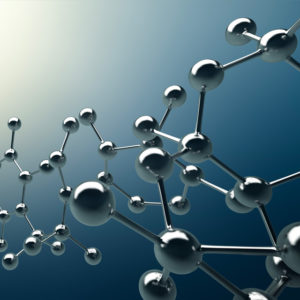## Refractrometry

Refractometry is an analytical method to measure the refractive index of a solid or liquid material. The refractive index is the ratio of the velocity of an electromagnetic wave in vacuum relative to its velocity in the material of interest. Refractive index can be measured between 1.30 and 1.71 at a wavelength of 589.3 nm, using an Abbe refractometer.

## Ideal Uses of Refractometry

• Liquids
• Glasses
• Plastics, polymer films
• Determination of the mass percentage of sugar in water
• Determination of the composition of binary mixtures## Strengths

• Fast measurement
• High accuracy

## Limitations

• Limited measurement range
• The refractive index can only be determined at one wavelength
• Sample must be light transmissive at the measurement wavelength used

## Refractometry Technical Specifications

Characteristics of Abbe refractometer:

• Obtained information: Refractive index and dispersion
• Sample types: Solids and liquids
• Sample size: Approx. 10×10 mm (glass polished surface)
• Measuring range: 1.30-1.71 nD
• Accuracy: 0.0002
• Temperature range: 0-150oC